# How to Calculate Torque of DC Motor?

As you are familiar with the principle of a DC motor or DC machine that when it is loaded either as a motor or as a generator, conductors of the rotor are carrying the current. To better understand the concept, imagine the conductors which are laying in the magnetic field which is present in the air gap.

Due to that magnetic field each of the conductor experiences an electromagnetic force. Obviously due to the impact of that force conductors which is laying near to the surface of the rotor and also having the common radius from the center of the rotor, the torque is produced. Since the torque produced is applied at the circumference of the rotor it causes the rotor to start rotating.

In order to understand the torque, we should refer to the explanation of Dr. Huge d Young where he states that the torque is the quantitative measure of the tendency of a force to cause a rotational motion, or a force to bring about a change in rotational motion. It is in fact the momentum of a force that produces or changes a rotational motion. The equation of torque is given by,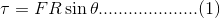Where, F is force in linear direction. R is radius of the object being rotated, and θ is the angle, the force F is making with R vector.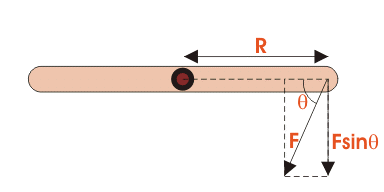Now if we talk about a DC motor which also works on the same principle since it is also a rotational machine. Understanding of the torque of DC motor is a very important in order to understand its operational parameters and its speed control etc. So we can say that in order to understand the running behavior and implications of any type of torque DC motor we must deeply look at the torque equation of a DC motor.

## Torque Equation of DC Motor

In order to have better understanding of torque motor equation below are given the basic circuit diagram of a DC motor. Let’s discuss, please see the diagram thoroughly.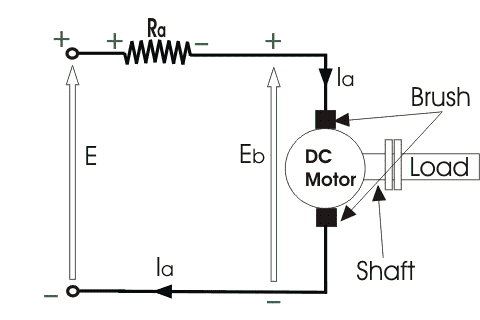Voltage equation of above circuit is given by:

Where:

E is the supply voltage

Eb is the back emf produced

Ia is armature current

Ra is armature resistance

Therefore, torque equation of DC motor becomes as below by multiplying both sides of above equation (2) by Ia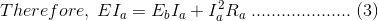Since Ia2 Ra is the power loss due to heating of the armature coil. True effective mechanical power to produce the desired torque of DC machine is given by,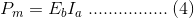The mechanical power Pm is related to the electromagnetic torque Tg as below: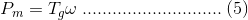Where ω is speed in rad/sec.

Now if we equate equations (4) & (5) above we get: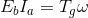To get more simplified shape of torque motor equation substitute in above equation Eb and ω by below values: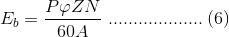Where:

P is no of poles

φ is flux per pole

Z is no. of conductors

A is no. of parallel paths

N is the speed of the D.C. motorSubstituting equation (6) and (7) in equation (4), we get: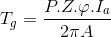Till this point of our exercise of finding the actual torque of a DC motor we have obtained the electromagnetic torque.

Next stage is to subtract all the mechanical and rotational losses from it to get the mechanical torque. So simplified form of the torque equation is given below:

Tm = Tg – mechanical losses i.eIt can be understood from above equation that the torque of a DC motor is constant for a particular machine at constant values of flux and armature current so the torque of the motor can be varied by making changes in flux φ and armature current Ia.

### Alternatively Understanding the DC Motor Torque

Refer below image of a dc motor.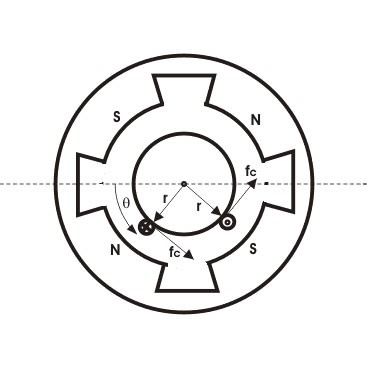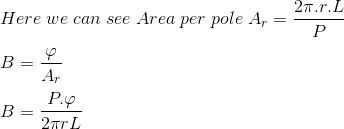Current / conductor Ic = Ia / A

Therefore, force per conductor: fc = BLIa/A

Now torque: Tc = fc.r = BLIa.r/AHence, the total torque developed of a DC machine is,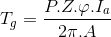This torque equation of DC motor can be further simplified as: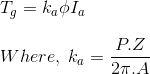Again we can see that the equation elaborates that torque is always constant for a particular DC motor, which proves that torque of any DC motor can be varied or changed by varying its flux φ and armature current Ia.

#### How To Control The Torque of a DC Motor

Hope you have got good idea about the torque machine equation. Now lets see a little about torque control of a DC motor.

The speed control of induction motor is done using direct torque control with four switch three phase inverter. The switching technique used is space vector modulation technique. The two phases are connected to the two legs of the inverter, while the third phase is connected to the mid point of the dc-bus voltage.

Stator flux linkage is estimated by integrating the stator voltages. Torque is estimated as a cross product of estimated stator flux linkage vector and measured motor current vector. The estimated flux magnitude and torque are then compared with their reference values.

## 2 thoughts on “How to Calculate Torque of DC Motor?”

1.atul says:

It’s actually a great and helpful piece of information.I am really thankful to the blog owner for help us by giving valuable study materials.

2.Victoria says:

I used to be recommended this web site by my cousin. I am now not certain whether or not this publish is written by him
as nobody else realize such distinct about my problem.
You’re wonderful! Thanks!